This post explains what an sPlot is. This well-known method was recently added to hep_ml library.

An sPlot is a way to reconstruct features of mixture components based on known properties of distributions. This method is frequently used in High Energy Physics.

## Simple example of sPlot

First we start from a simple (and not very useful in practice) example.

Assume we have two types of particles (say, electrons and positrons).

The distribution of some characteristic is different for them (let this be the px — a momentum projection).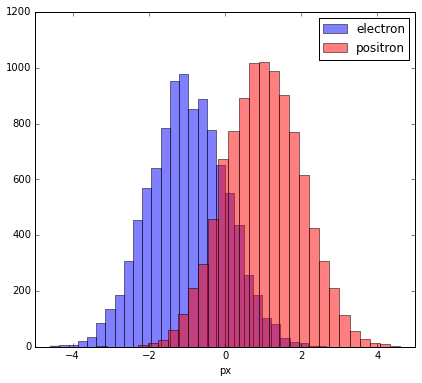## Observed distributions

Picture above shows how this distribution should look like, but due to inaccuracies during classification we will observe a different picture, and this is important.

Let’s assume that with a probability of 80% a particle is classified correctly (and also assume px is not used during classification).

When we plot the distribution of px for particles which were classified as electrons or positrons, we see that distributions are distorted. We lost the original shapes of distributions.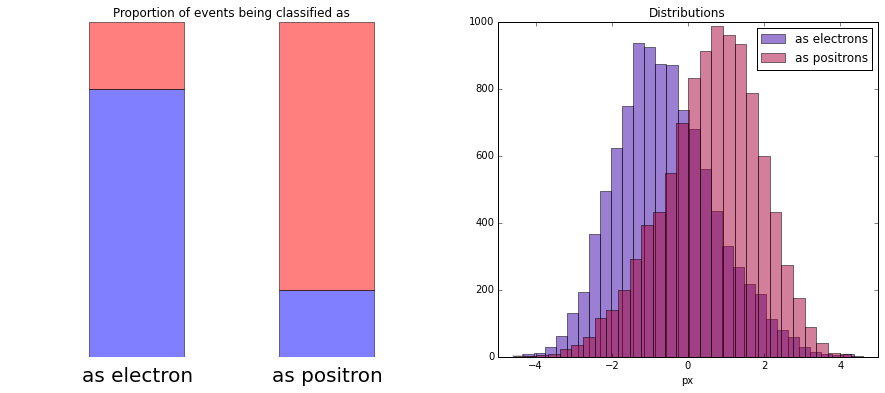## Applying sWeights

Think of it in the following way: there are 2 bins. First bin contains 80% of electrons and 20% of positrons. And visa versa in the second bin.

To reconstruct the initial distribution, one can plot the histogram where each event from the first bin has weight 0.8, and each event from the second bin has weight -0.2. These numbers are called sWeights.

In other words, let’s say we had 8000 $e^{-}$ + 2000 $e^{+}$ in first bin and 8000 $e^{+}$ + 2000 $e^{-}$ ($e^-, e^+$ are electron and positron). After summing with introduced sWeights:

$$( 8000 e^{-} + 2000 e^{+} ) \times 0.8 + ( 2000 e^{-} + 8000 e^{+} ) \times (- 0.2) = 6800 e^{-}$$

Positrons with positive and negative weights compensated each other, and we got “pure electrons”.

At the moment we ignore the normalization of sWeights (since right now only the shape of distributions is of interest).### Compare

Let’s compare reconstructed distribution for electrons with original:# More complex case

In the case when we have only two ‘bins’ things are simple and straightforward.

But when there are more than two bins, the solution is not unique. There are many appropriate combinations of sWeights, which one to choose (like in example below with 3 bins)?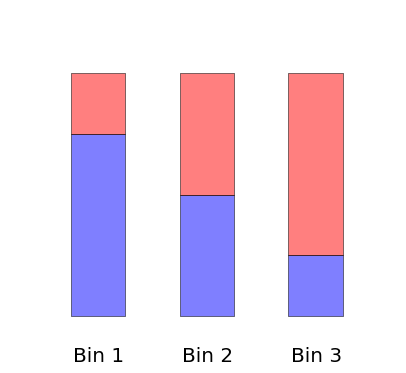But things are more complex in practice. We have not bins, but continuous distributions (which can be treated as many bins).

Typically this is a distribution over mass. By fitting the mass we are able to split the mixture into two parts: signal channel and everything else.

# Building sPlot over mass

Let’s show how this works. First we generate two fake distributions (signal and background) with 2 variables: mass and momentum.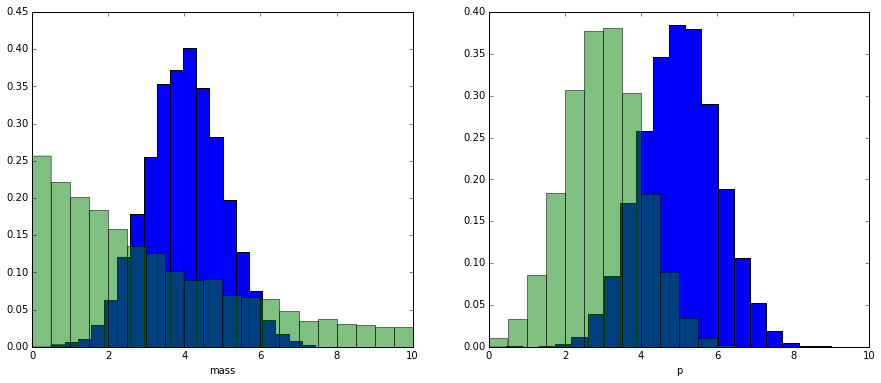### Of course we don’t have labels which events are signal and which are background.

And we observe the mixture of two distributions: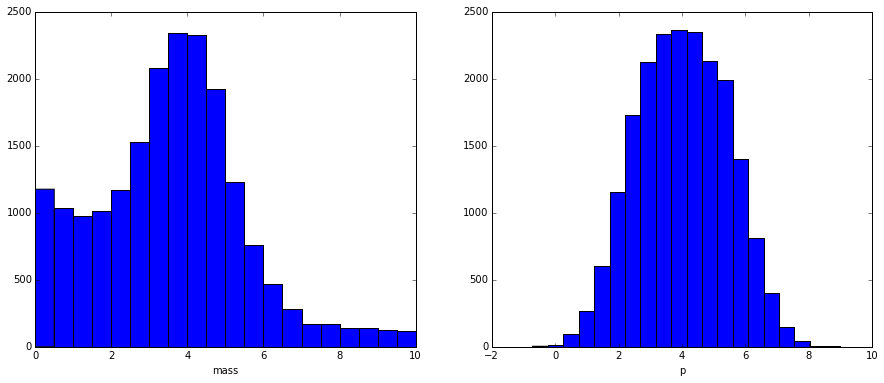### We have no information about real labels

But we know a priori that the background is distributed as an exponential distribution and signal as a gaussian (more complex models can be met in practice, but idea is the same).

After fitting the mixture (let me skip this process), we get the following result: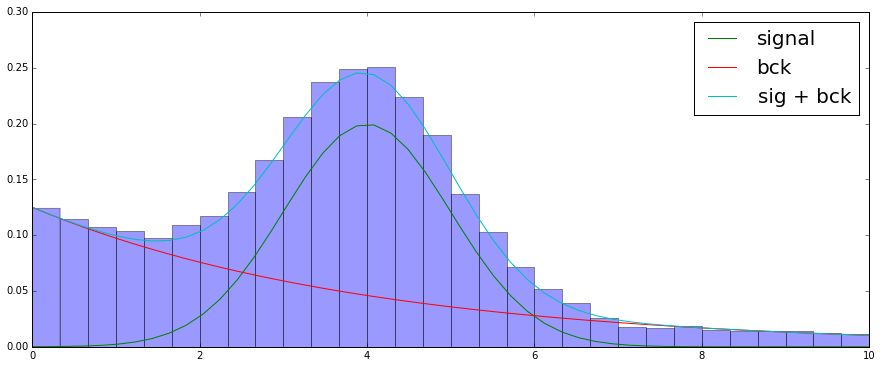## Fitting doesn’t give us information about real labels

But it gives information about probabilities, which allows us to estimate the number of signal and background events within each bin.

We won’t use bins, but instead we’ll compute for each event probability that it is signal or background (this probability is computed from the mass. Make sure you see the connection with previous plot):## Applying sPlot

sPlot converts probabilities to sWeights, using the implementation from hep_ml:

As you can see, there are also negative sWeights, which are needed to compensate the contributions of other class (remember that in the first example we needed negative weights).## Using sWeights to reconstruct initial distribution

Let’s check that we achieved our goal and now we can reconstruct momentum distribution for signal and background using sWeights: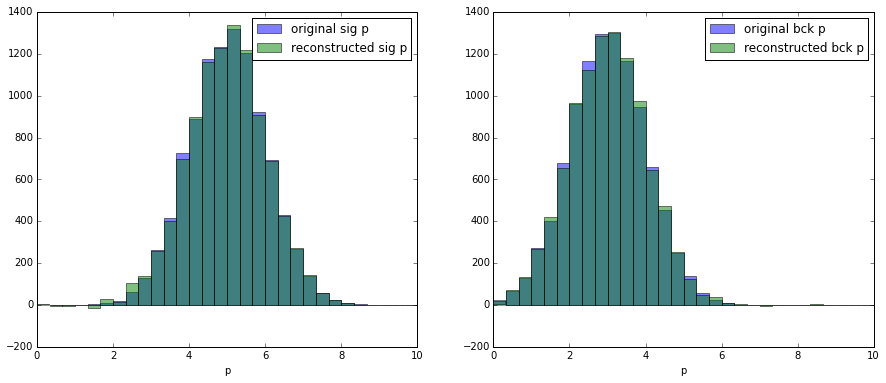## Important requirement of sPlot

Reconstructed variable (i.e. $p$ or lifetime or flight distance) and splotted variable (i.e. mass) shall be statistically independent within each class.

Read the line above again. Reconstructed and splotted variable are correlated, but when you consider only signal they are independent!

as a demonstration why this is important let’s use sweights to reconstruct the mass (obviously the mass is correlated with the mass):# Derivation of sWeights

Now, after we seen how this works, let’s derive the formula for sWeights.

The only information we have from fitting over the mass is $p_s(x)$, $p_b(x)$ which are probabilities of event $x$ to be signal and background.

Our main goal is to correctly reconstruct a histogram. Let’s reconstruct the number of signal events in a particular bin. We introduce unknown $p_s$ and $p_b$ — probability that signal or background event will be in the named bin (that’s just proportions so far).

(Since mass and reconstructed variable are statistically independent for each class, $p_s$ and $p_b$ do not depend on mass.)

The mathematical expectation should be obviously equal to $p_s N_s$, where $N_s$ is total amount of signal events available from fitting.

Let’s also introduce random variable $1_{x \in bin}$, which is 1 iff event $x$ lies in selected bin.

The estimate for number of signal event in bin is equal to: $$X = \sum_x sw_s(x) \; 1_{x \in bin},$$ where $sw_s(x)$ are sPlot weights and are subject to find.

## First main property of sweights

Property 1. We expect estimate to be unbiased

$$\mathbb{E} \, X = p_s N_s$$

Corollary Let’s understand what this means for sPlot weights.

$p_s N_s = \mathbb{E} \, X = \sum_x w_s \; \mathbb{E} \, 1_{x \in bin} = \sum_x w_s \; (p_s p_s(x) + p_b p_b(x))$

In the line above I used the assumption that variables are statistically independent for each class.

Since the previous equation should hold for all possible $p_s$ and $p_b$, we get two equalities:
$p_s N_s = \sum_x sw_s(x) \; p_s p_s(x)$
$0 = \sum_x sw_s(x) \; p_b p_b(x)$

After reduction:
$N_s = \sum_x sw_s(x) \; p_s(x)$
$0 = \sum_x sw_s(x) \; p_b(x)$

This way we can guarantee that average contribution of background is 0 (expectation is zero, but observed number will not be zero due to statistical deviation), and the expected contribution of signal is $N$

## Under assumption of linearity:

assuming that sPlot weight can be computed as a linear combination of conditional probabilities:

$sw_s(x) = a_1 p_b(x) + a_2 p_s(x)$

We can easily reconstruct those numbers, first let’s rewrite our system:

$\sum_x (a_1 p_b(x) + a_2 p_s(x)) \; p_s(x) = 0$
$\sum_x (a_1 p_b(x) + a_2 p_s(x)) \; p_b(x) = N_{sig}$

$a_1 V_{bb} + a_2 V_{bs} = 0$
$a_1 V_{sb} + a_2 V_{ss} = N_{sig}$

Where $V_{ss} = \sum_x p_s(x) \; p_s(x)$, $V_{bs} = V_{sb} = \sum_x p_s(x) \; p_b(x)$, $V_{bb} = \sum_x p_b(x) \; p_b(x)$

Having solved this linear equation, we get needed coefficients (as those in the paper)

NB. There is minor difference between $V$ matrix I use and $V$ matrix in the paper.

## Minimization of variation

Previous part allows one to get the correct result. But there is still no reason for linearity given, we just assumed this..

Apart from having a correct mean, we should also minimize variation of any reconstructed variable. Let’s try to optimize it

$$\mathbb{V}\, X = \sum_x sw_s(x)^2 \; \mathbb{V}\, 1_{x \in bin} = \sum_x sw_s(x)^2 \; (p_s p_s(x) + p_b p_b(x))(1 - p_s p_s(x) - p_b p_b(x))$$

A bit complex, isn’t it? Instead of optimizing such a complex expression (which is individual for each bin), let’s minimize it’s uniform upper estimate

$$\mathbb{V}\, X = \sum_x sw_s(x)^2 \; \mathbb{V}\, 1_{x \in bin} \leq \sum_x sw_s(x)^2$$

so if we are going to minimize this upper estimate, we should solve the following optimization problem with constraints:
$\sum_x sw_s(x)^2 \to \min$
$\sum_x sw_s(x) \; p_b(x) = 0$
$\sum_x sw_s(x) \; p_s(x) = N_{sig}$

Let’s write lagrangian of optimization problem:

$$\mathcal{L} = \sum_x sw_s(x)^2 + \lambda_1 \left[\sum_x sw_s(x) \; p_b(x) \right] + \lambda_2 \left[\sum_x sw_s(x) \; p_s(x) - N_{sig} \right]$$

After taking derivative with respect to $sw_s(x)$ we get the equality:

$$0 = \dfrac{\partial \mathcal{L}}{\partial \; sw_s(x)} = 2 sw_s(x) + \lambda_1 p_b(x) + \lambda_2 p_s(x)$$

which holds for every $x$. Thus, after renaming for convenience $a_1 = - \lambda_1 / 2,$ $a_2 = - \lambda_2 / 2,$ we confirmed linear dependency.

### Statistical independence

The main assumption we used here is that distribution inside each bin is absolutely identical.

In other words, we stated that there is no correlation between the index of bin and the reconstructed variable. Remember that bin corresponds to some interval in mass, and finally we get:

reconstructed variable shall not be correlated with mass variables (or any other splotted variable)

# Conclusion

1. sPlot allows reconstruction of some variables.
2. the only information used is probabilities taken from fit over variable. If fact, any probability estimates fit well.
3. the source of probabilities should be statistically independent from reconstructed variable (for each class!).
4. mixture may contain more than 2 classes (this is supported by hep_ml.splot as well)

## Sources and code

The code for this post may be found at hep_ml repository.

A very close explanation was written by Michael Schmelling. Updated version which is publicly available.

Many thanks to Konstantin Schubert for proof-reading this post.